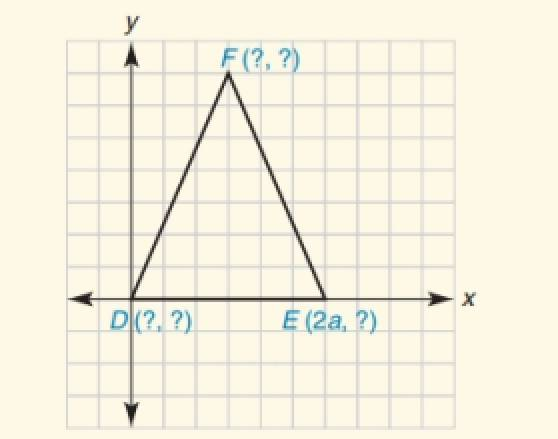Chapter 10.CT, Problem 13CT### Elementary Geometry for College St...

6th Edition
Daniel C. Alexander + 1 other
ISBN: 9781285195698

#### Solutions

Chapter
Section### Elementary Geometry for College St...

6th Edition
Daniel C. Alexander + 1 other
ISBN: 9781285195698
Textbook Problem
1 views

# Using as few variables as possible, state the coordinates of each point if △ D E F is isosceles with ≅D E F is an isosceles triangle with ≅ D (       ,             _ ) , E (       ,             _ ) ,     F (       ,             _ ) .

To determine

To find:

The missing coordinates for the vertices.

Explanation

The given figure is shown below.

From the figure, the following observations are made.

Since, the vertex D is at the origin.

The coordinates of D0, 0.

Now, consider the vertex E.

Here, x-coordinate is given as 2a.

In the E vertex, observe where it lies on y- axis.

Since, it is at 0, the vertex E has the coordinates 2a, 0.

The distance from D to E in the x- axis is 2a.

F lies midway between D and E

### Still sussing out bartleby?

Check out a sample textbook solution.

See a sample solution

#### The Solution to Your Study Problems

Bartleby provides explanations to thousands of textbook problems written by our experts, many with advanced degrees!

Get Started

#### Find more solutions based on key concepts